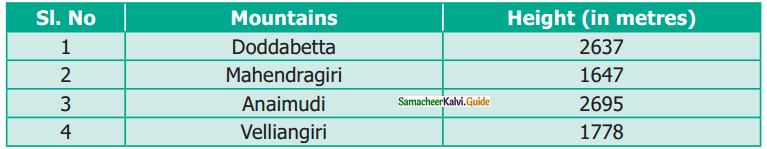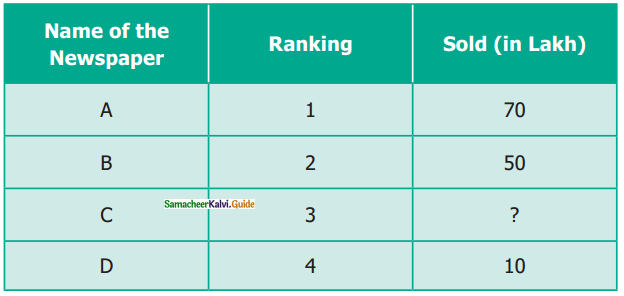Students can download Maths Chapter 1 Numbers Ex 1.2 Questions and Answers, Notes, Samacheer Kalvi 6th Maths Guide Pdf helps you to revise the complete Tamilnadu State Board New Syllabus, helps students complete homework assignments and to score high marks in board exams.

## Tamilnadu Samacheer Kalvi 6th Maths Solutions Term 1 Chapter 1 Numbers Ex 1.2

Question 1.
Fill in the blanks with > or < or =.

1. 48792 ………. 48972
2. 1248654 ……… 1246854
3. 658794 ……… 658794

Solution:

1. <
2. >
3. =Question 2.
Say True or False.

1. The difference between the smallest number of seven digits and the largest number of six digits is 10.
2. The largest 4 digit number formed by the digits 8, 6, 0, 9 using each digit only once is 9086
3. The total number of 4 digit number is 9000

Solution:

1. False
Hint: 1000000 – 999999 = 1
2. False
Hint: 9999 – 999 = 9000
3. True

Question 3.
Of the numbers 1386787215, 137698890, 86720560, which one is the largest? Which one is the smallest?
Solution:
We know that the number with more digits is greater.
The greatest number is 1386787215
The smallest number is 86720560Question 4.
Arrange the following numbers in the descending order:
128435, 10835, 21354, 6348, 25840
Solution:
128435 > 25840 > 21354 > 10835 > 6348

Question 5.
Write any eight-digit number with 6 in ten lakhs place and 9 in ten-thousandth place.
Solution:
76594231
86493725

Question 6.
Rajan writes a 3-digit number, using the digits 4, 7, and 9. What are the possible numbers he can write?
Solution:
974, 947, 479, 497, 749, 794Question 7.
The password to access my ATM card includes the digits 9, 4, 6, and 8. It is the smallest 4 digit even number. Find the password of my ATM card.
Solution:
Given digits are 9, 4, 6, and 8.
The smallest number with these digits is 4689 Given that it is an even number.
It may be 4698.
So password of the ATM card is 4698.

Question 8.
Postal Index Number consists of six digits. The first three digits are 6, 3, and 1. Make the largest and the smallest Postal Index Number by using the digits 0, 3, and 6 each only once.
Solution:
Largest Postal Index Number = 631603
Smallest Postal Index Number = 631036Question 9.
The heights (in meters) of the mountains in Tamil Nadu are as follows:(i) Which is the highest mountain listed above?
(ii) Order the mountains from the highest to the lowest.
(iii) What is the difference between the heights of the mountains Anaimudi and Mahendragiri?
Solution:
(i) Anaimudi (2695 m)
(ii) Anaimudi, Doddabetta, Velliangiri, Mahendragiri
(iii) 2695 m – 1647 m = 1048 m

Objective Type Questions

Question 10.
Which list of numbers is in order from the smallest to the largest?
(a) 1468, 1486, 1484
(b) 2345, 2435, 2235
(c) 134205, 134208, 154203
(d) 383553, 383548, 383642
Solution:
(c) 134205, 134208, 154203Question 11.
The Arabian Sea has an area of 1491000 square miles. This area lies between which two numbers?
(a) 1489000 and 1492540
(b) 1489000 and 1490540
(c) 1490000 and 1490100
(d) 1480000 and 1490000
Solution:
(a) 1489000 and 1492540

Question 12.
The chart below shows the number of newspapers sold as per the Indian Readership Survey in 2018. Which could be the missing number in the table?(a) 8
(b) 52
(c) 77
(d) 26
Solution:
(d) 26